Courses

# General EMF And Torque In DC Machines Electrical Engineering (EE) Notes | EduRev

## Electrical Engineering (EE) : General EMF And Torque In DC Machines Electrical Engineering (EE) Notes | EduRev

The document General EMF And Torque In DC Machines Electrical Engineering (EE) Notes | EduRev is a part of the Electrical Engineering (EE) Course Electrical Machines.
All you need of Electrical Engineering (EE) at this link: Electrical Engineering (EE)

General EMF and Torque in DC Machines
In the last class, we have seen that the cross section of a direct current machine.

(Refer Slide Time: 0031)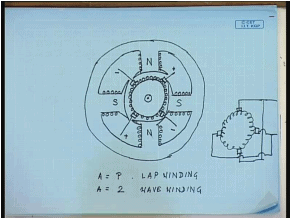Can be drawn somewhat like this, it has a stator core to which holes are connected stationery poles field poles. In general a dc machine can have more than two poles. The poles are fitted with pole shoes, which cover the most of the air gap part and carries winding. These windings are electrically excited with dc current, such that alternate north and south poles are generated. The armature the rotor carries the armature. There is mounted on a shaft axial shaft the connection to the rotor are obtained by means of commutators and brush segments. The brushes are normally pressed what we call the inter-polar region?

That is it connects to the conductor that are at the inter-polar region. This is plus plus minus minus the brushes divide the armature is a closed winding the brush divide the armature in several parallel path. The closed winding of the armature can be schematically represented like this, and the brushes here the positive creases are connected together, the negative are also connected together and two terminals are finally brought out. However, the brushes divide the whole armature winding into a number of parallel paths number of parallel paths a is equal to the number of poles of the dc machine for lap winding and the number of parallel paths a equal to 2 for wave winding.

Now, when such an arrangement is rotated inside the stator, this armature is rotated inside the stator an EMF is induced in the armature coils, and across the brushes as the coils are serious connected between brushes as well as if a current is allowed to flow through these conductors by either connecting an external resistance and rotating the machine in which case it access as generator or by supplying the armature from an external voltage source in which case it access a motor. A torque is also produced. In this class we will try to find put the expressions of this generated emf and the generated torque as functions of different machine parameters for this purpose, let us take what we call a developed view of this cross section? Developed view is nothing but cutting the cross sectioned diametrically and unfolding it in a straight line.

(Refer Slide Time: 06:40)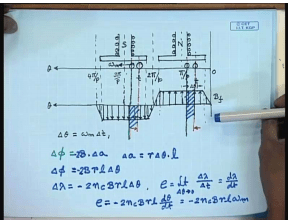If we do that exercise along these axes and fold it open then it will look somewhat like this. Thus this is my position 0. There are p number of poles if this is the inter polar region then the axis of the first north pole will be at a position pi by p. the next inter polar region will come at 2 pi by p. Next pole will come at 3 pi by p. And, then you will come back to the original position and the next polar region inter-polar region will be at 4 pi by p. Since, if you had a pole pair n pair of poles the action due to a pair of poles is identical for all pole pairs will concentrate only on the behaviour of the machine under one set of pole pairs.

So, our diagram see here let us say this is the north pole, and this is the south pole. The poles are fitted with pole shoes which cover most of the armature surface therefore. you can draw the pole shoes somewhat like this. So, this is north pole, this is south pole the flux density waveform produced by the field winding can be approximated by a flat top for which goes to 0 in the at the inter polar region. In this region flux is going from the pole to the armature, which we call positive flux and denote by these arrows. And, this is a negative flux which flows from the armature to the south pole and denoted by these negative arrows.

So, this is the flux density waveform due to the field winding alone. Now, consider at any time a coil on the armature full pitch coil by that we mean that if one end of that coil is under north pole the other end will be under south pole. Let this be the position of the coil at time T and let the armature along with this coil be moving in this direction with an angular velocity of omega m, then after a short time T plus delta T, this coil will move by an angle delta theta. This angle will be delta theta, what will be delta theta? Delta theta equal to omega n into delta T. Now, let us look at the change in flux leakage of this coil delta lambda.

At time T the flux passing through the coil can be found by integrating the flux density waveform between these two limits, and at time T plus delta T the flux density waveform flux, then flux linkage can be found by integrating the flux density open between these two lines. However, let us find out the change in flux leakage or rather change in flux first delta phi. The change in flux delta phi if we try to find out we see that the flux passing through this area. Positive flux passing through this area has gone out of the coil as well as some amount of negative flux has been included and from the symmetry of the flux density waveform they are equal.

How much is the flux contained is this hast portion it can be found from the flux density waveform and the area the area of that the armature area due to this angle delta theta delta a equal to the arc length r into delta theta into the length of the armature. So, delta phi equal to minus two B into delta a, that is delta phi equal to Br l delta theta. So, and 2 times this and that is in the negative direction. So, this is minus 2 times b r l delta theta and the change in flux linkage delta lambda equal to number of turns in this coil let us denote it by n c. So, delta lambda equal to minus 2 n c b r l delta theta. So, the magnitude of the emf induced in this coil at the instant t is given by e equal to limit delta theta tending to 0 delta lambda by delta t. That is equal to d lambda d t. So, e equal to minus 2 n c b r l d theta d t that is minus 2 n c b r l omega m. So, we see that the voltage induced a coil at a given position theta is proportional to the flux density at that position and the rotational speed of the machine.

(Refer Slide Time: 18:44)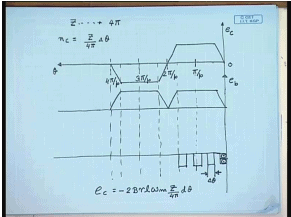So, we can draw the waveform of the induced voltage in that particular coil as it moves along the armature surface. Since, 0 this is pi by p this is 2 pi by p this is 3 pi by p this is 4 pi by p, as the coil moves along this direction the emf induced has a similar waveform as that of the flux density waveform. That is where theta is the position of the first end of the coil. Now, this is to be understood that we do not see this waveform on the brush. Since, the coils are connected to the brush through commutators, let us try to visualize the situation the brushes are placed at the inter-polar region. Therefore, this coil will connect to the brush first when it is at position 0 and the induced voltage in the coil is 0, and if this is the only coil then the commutator segment spends half the armature periphery, therefore for half the cycle it will remain connected to one commutator segment and then for the rest half it will connect to the other commutator segment, hence in effect the voltage induced in the brush voltage in at the brush will be the rectified waveform of the voltage induced in the coil.

This is the voltage induced in the coil this is the voltage in the brush, across the brush. This will be would have been the voltage across the brush had there been only one coil on the armature, but now consider a practical dc machine where there will be large number of such coils. For example, if we look at the armature surface, this contains slots. and dc machines usually have double layer. So, you each slot there will be two coil lengths. So, and if we assume that one of this slot width of denote the width of the slot by delta theta, then the number of turns n c in a coil can be represented in terms of delta theta as follows.

Let there be total conductor in the armature these are uniformly distributed over the armature periphery. So, and the armature is double layer. So, the z numbers of conductor are distributed over a mechanical angle of 4 pi. So, the number there is the number of turns in a coil that is conductor in a coil is n c, So n c can be written as z divided by 4 pi into delta theta hence the voltage induced in a coil e c equal to minus 2 B r l omega m z by 4 pi d theta, that is the voltage induced in a single coil. Now, it is to be understood that between two brushes. There are number of coils connected in parallel. In fact, all the coils that are in the span 2 pi by a brushes are set apart by an angle of the number of conductors between two opposite polarity brushes is given by 2 pi by a. The number of coils connected in series between two brushes is given by 2 pi by a.

(Refer Slide Time: 25:49)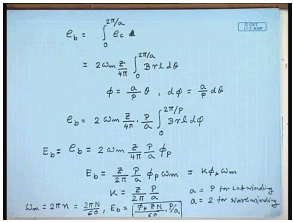Therefore the induced voltage between the brushes can be written as integral e b in a practical d c machine can be written as integral 0 to because one of the brush will be at the inter polar region 0 the other will be at 2 pi by p, but the number of coils connected in series between these two brushes will be 2 pi by a, where a is the number of parallel paths. So, 2 pi by a e c d theta, e c which is equal to 2 omega n z by 4 pi integral 0 to 2 pi by a B r l d theta. Now, let us do a change of variable as follows it has defined phi equal to a by p theta.

Therefore, d phi equal to a by p d theta. So, e b can be written as 2 omega m z by four pi into 2 by a integral 0 to 2 pi by p B r l d phi. This term under integration can be easily identified as the total flux passing through the north pole, hence this is termed the flux per pole of phi p therefore, the simple formula of induced voltage across two brushes is given by. So, this is also the terminal voltage of the dc machine or the generated back emf in the dc machine because the voltage is measured across the brushes. So, the back emf expression is given by e b equal to z divided by 2 pi p by a phi p omega n this is sometimes written as k phi p omega m, where k is the back e m f constant as given by k equal to z by 2 pi p by a.

Now, a equal to p for lap winding, and a equal to 2 for wave winding. There is a slightly more slightly different formula for this e b also if we write omega m in terms of rpm then omega m equal to 2 pi into n, where n is the rotational speed of the dc machine rotor in rps or equal to 2 pi n by 60, where the capital n is the rotational speed in rpm. So, e b can be written as phi p z by phi p z n by 60 into p by a, this formula is also very much in use. So, we have seen that how to find out the induced emf in a dc machine from the first principle. Now, let us find out the torque for that.

(Refer Slide Time: 32:06)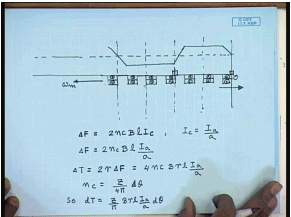We again go back to the original diagram of the developed diagram, the flux density waveform due to field winding. We have mentioned is trapezoidal that is of this shape and the armature contains slots and coils in the slots in double layer. We have seen that if this armature is moving at a speed omega m in this direction. Then, there will be a induced voltage in this coils, what will be the direction of the induced voltage from faradays principle? We can find say that the induced emf will be in such a direction that if a current is allowed to flow in the coils it will oppose the change of flux linkage.

Now, we have seen that due to the motion the flux linkage change is in the negative direction that is flux link positive flux linkage tends to decrease, so the current will flow in this coil in a such a fashion that this decrease in the flux linkage is compensated that is the currents in these coils will produce positive flux in this direction. So, what will be the direction of current flow? it has to be understood that the positive flux means flux flowing from the field to the armature definitely, then the current flowing in this coils will be of dot polarity and current the other side of the brush will have cross polarity due to the induced emf. Now, we also know that if there is a current carrying conductor under a magnetic field, then the conductors experience a force and on the flemming’s left hand rule. We can find out the direction of the force in this case the direction of the force will be opposite to motion.

We can also find out the magnitude of this force acting on some conductor, let us say one coil delta f will be equal to the number of conductors at any given position which due to double layer winding is 2 n c. So, this will be 2 n c and then B into l and into I c that is the current flowing through this conductors. Now if the armature has a number of parallel paths, then I c equal to armature current I a by a there put delta f equal to 2 n c B l I a by a, now let us look at the original diagram of the dc machine the conductors here are experience a force of delta f in the direction opposite to the motion.

Similarly the conductors diametrically opposite are also experiencing a force and again in the direction opposite to the motion, so the torque produced by this conductor sets that these two sets of conductor is given by delta T equal to 2 into r into delta f equal to four n c B r l I a by a. However, we have found out n c equal to z by 4 pi into d theta. So, d T equal to z by pi b r l I a by a into d theta. Now, we know that the total torque in order to find the total torque will have to add the torque produced by individual coils between two brushes, because the rest of the coils will, now be taken care of by other sets of.

(Refer Slide Time: 40:57)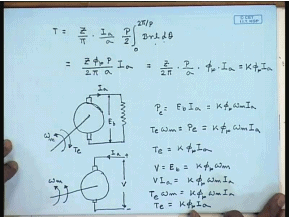So, there are between two brushes you will have to integrate them. So, torque T equal to by pi into I by I a by a you have to integrate over 1 pole pitch that is integral 0 to 2 pi by p b r l d theta. However, there are p by 2 such pole pairs which is generating the torque this is the torque produced by 1 pole pair. So, the total torque or in order to get total torque this has to be multiplies by p by 2. So, the total torque expression will this we again identify as flux pair pole. So, this will be z phi p by 2 pi a I a equal to z by 2 pi into p by a into phi p into I a, this is again can be written as k phi p I a, where k is the torque constant and we found that a torque constant is same as the back emf constant found during calculation of the induced emf.

This can also be shown from the power balance. Let us say this is the armature with the brushes with an external resistance connected and being rotated in the direction with a speed of omega n let the current here be I a if we assume no loss in the machine, that is if we assume armature resistance of the machine to be 0, then the power produced by the generator equal to e b into I a, the e v equal E b equal to k phi p omega m I a. The electrical power produced must be equal to the mechanical power input, so in order to rotate this armature some amount of mechanical work has to be done that is it has to be rotated against a generated torque T e.

Therefore T e omega n should be equal to P e equal to k phi p omega n I a or T e equal to k phi p I a, which is same as what we have obtained for as the torque expression from the first principle. This we have shown when the machine works as a generator, however the same conclusion will be arrived if we consider a motor mode of operation. In the motor mode of operation the only change will be the direction the armature terminals will be connected to an external source of voltage v and if we neglect the armature resistance then in steady state v equal to E b equal to k phi p omega m therefore, electrical power input to the machine is v I a equal to k phi p omega m I a.

This must be same as the mechanical work done where direction of torque will also now change because the direction of current has changed, so T e omega m should be equal to k phi p omega m I a or torque in the motor mode will also be given by k phi p I a. Therefore, we have seen that the dc machine, when the armature rotates under the influence of field poles field flux a emf is induced across the brushes the magnitude at the e m f is proportional to the flux produced by the field poles that is flux pair pole phi is the and the rotational speed of the armature in the same manner.

We have shown that if a current is allowed to flow in the armature conductors either by connecting a load in the generating mode or by connecting the armature to a separate voltage source to make it run as a motor then an electromagnetic torque is generated the magnitude wave is again proportional to the flux per pole and the armature current in the armature circuit. For the motoring mode the current is injected into the armature by an external circuit, so that electrical power is input to the machine, and hence the generated electromagnetic torque will be in a direction which will assist motion that is mechanical work will be done.

So, the direction of electromagnetic torque will be in the same direction as the that of rotation that it will assist rotation, and hence electrical power input will be converted to mechanical power output. The reverse will happen in the generating mode that is if a load is connected then the current flowing through the armature winding would try to stop the motor that is the electromagnetic torque produced would be in a direction which is opposite to the direction of rotation, however if the flux per pole and the magnitude of the armature currents are same in the motoring, and in the generating mode, then the magnitude of electrical torque produced will be given by the same formula that is k phi p I a both in motoring and in generating mode, similarly the induced voltage either in the motoring mode or in the generating mode will be given by the formula k phi p omega m.

(Refer Slide Time: 50:23)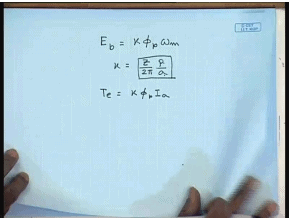So, whether the machine operates as a motor or as a generator the formula for induced voltage in a dc machine is given by E b equal to k phi p omega m, where k equal to z by 2 pi p by a, x is the total number of armature conductor p is the number if poles and a is the parallel path and electromagnetic torque is given by k phi p I a where k has the same expression .

Thank you.

Offer running on EduRev: Apply code STAYHOME200 to get INR 200 off on our premium plan EduRev Infinity!

61 docs|20 tests

,

,

,

,

,

,

,

,

,

,

,

,

,

,

,

,

,

,

,

,

,

;